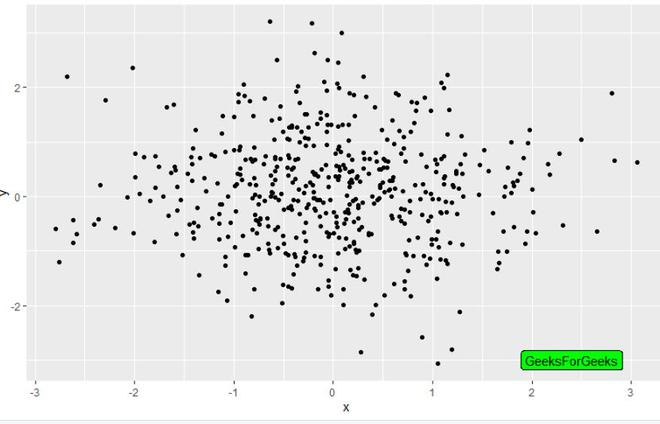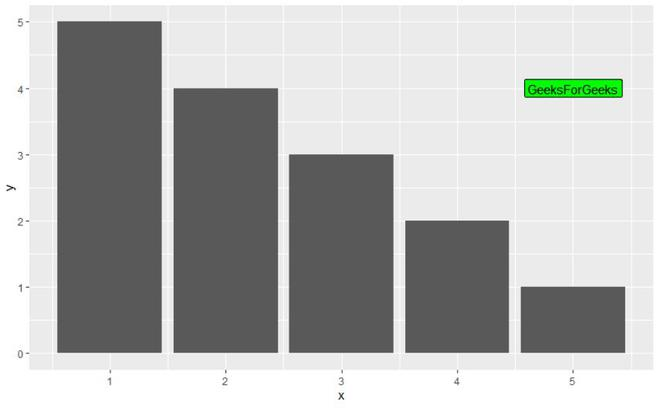Related Articles

# Change the Background color of ggplot2 Text Label Annotation in R

• Last Updated : 17 Jun, 2021

In this article, we will be looking at the approach to change the background color of ggplot2 text label Annotation in the R programming language.

This can be done using the geom_label() function using its fill argument. The user needs to first install and import the ggplot2 package in the R console, and then call the goem_label() function to add the label in the label argument and fill argument of this function to add the background color the created labels in the R programming language.

This function text geoms are useful for labeling plots. They can be used by themselves as scatterplots or in combination with other geoms.

Syntax:

geom_label(mapping = NULL,data = NULL, stat = “identity”, position = “identity”, …, parse = FALSE, nudge_x = 0, nudge_y = 0, label.padding = unit(0.25, “lines”), label.r = unit(0.15, “lines”), label.size = 0.25, na.rm = FALSE, show.legend = NA, inherit.aes = TRUE)

Example1:

## R

 `library``(``"ggplot2"``)`` ` `gfg_data=``data.frame``(x=``rnorm``(500),y=``rnorm``(500))``gfg_plot <- ``ggplot``(gfg_data, ``aes``(x, y)) + ``geom_point``()``gfg_plot+``geom_label``(``aes``(x = 2.4, y = -3,label = ``"GeeksForGeeks"``),``                    ``fill = ``"green"``)`

Output:Example2:

## R

 `library``(``"ggplot2"``) `` ` `gfg_data<-``data.frame``(x=``c``(1,2,3,4,5),y=``c``(5,4,3,2,1))``gfg_plot<-``ggplot``(data=gfg_data, ``aes``(x, y)) +``  ``geom_bar``(stat=``"identity"``)``gfg_plot + ``geom_label``(``aes``(x = 5, y = 4,label = ``"GeeksForGeeks"``),``                      ``fill = ``"green"``)`

Output:My Personal Notes arrow_drop_up